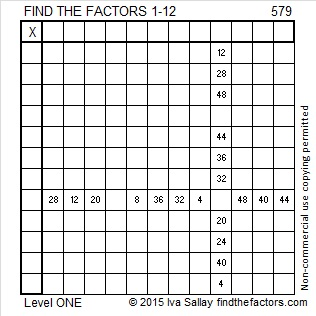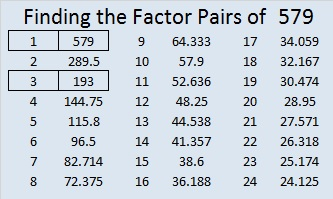# 579 and Level 1

579 is the hypotenuse of the Pythagorean triple 285-504-579. Which of 579’s factors is the greatest common factor of those three numbers?

Last week someone googled “find,the least 6 digit which has 173 factor” and arrived at Findthefactors.com. Here how to find the answer: Divide 100,000 by 173 and get 578.03 approximately. Round that answer up to 579. Multiply 579 by 173 and get 100167, the smallest 6-digit number that has 173 as a factor.Print the puzzles or type the solution on this excel file: 12 Factors 2015-08-10

—————————————————————————————————

• 579 is a composite number.
• Prime factorization: 579 = 3 x 193
• The exponents in the prime factorization are 1 and 1. Adding one to each and multiplying we get (1 + 1)(1 + 1) = 2 x 2 = 4. Therefore 579 has exactly 4 factors.
• Factors of 579: 1, 3, 193, 579
• Factor pairs: 579 = 1 x 579 or 3 x 193
• 579 has no square factors that allow its square root to be simplified. √579 ≈ 24.0624188—————————————————————————————————This site uses Akismet to reduce spam. Learn how your comment data is processed.